# Calculate Pi by Throwing Hotdogs !

| 1 Comment | 0 TrackBacks

Recently I started teaching my 10 year old son about Pi, its relationship to Circles, how and what Pi can be used for and some methods to determine its value.

During this I stumbled on an article about How to calculate Pi by Throwing Frozen Hotdogs.
http://m.wikihow.com/Calculate-Pi-by-Throwing-Frozen-Hot-Dogs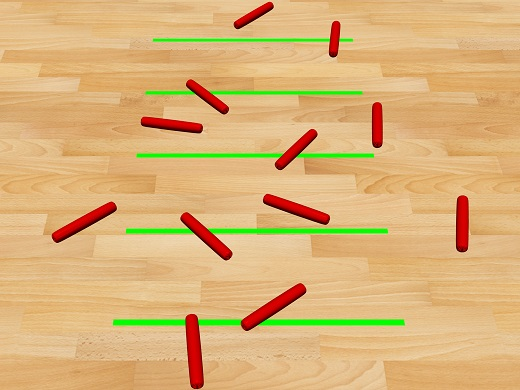This is more correctly known as the Buffon's Needle.

Buffon's Needle is a technique that uses the probability of throwing a needle onto a grid where the grid is spaced at the length of the needle. From this an estimate of Pi can be made.
There is mathematics behind this and I am not even going and try and explain why this works, but for those interested, you can read about it here.
http://en.wikipedia.org/wiki/Buffon%27s_needle

Obviously this was crying out for an Excel implementation.
I mean, "Who doesn't want to throw hotdogs with Excel!"

The Setup

The technique of estimating Pi by throwing hotdogs onto a grid involves marking out a grid on the floor with grid lines spaced equivalent to the length of the hotdog.

You then randomly throw hotdogs onto the grid and tally up the number of hotdogs that cross a grid line.

Pi is then estimated as the Total number of Hotdogs /Number of Hotdogs that cross a grid line/2.

In Excel, I originally thought of using a chart, but adding new series to a chart requires VBA.
I also wanted to be able to throw many thousands of hotdogs and most chart types have a limit of 255 series, although the number of points can be much greater than 255. I also needed the ability to rotate each hotdog individually.

I considered doing it all in VBA and drawing hotdogs on a UserForm, but that just seems too non-Excel.

I finally settled on using a Worksheet Range as the Grid, where I could obviously set the Row Spacing as the Hotdog's length.

I decided on a hotdog length of 50. This allowed for 10 Rows in the Grid and left room for user controls and result monitoring charts on a worksheet that would fit on most displays.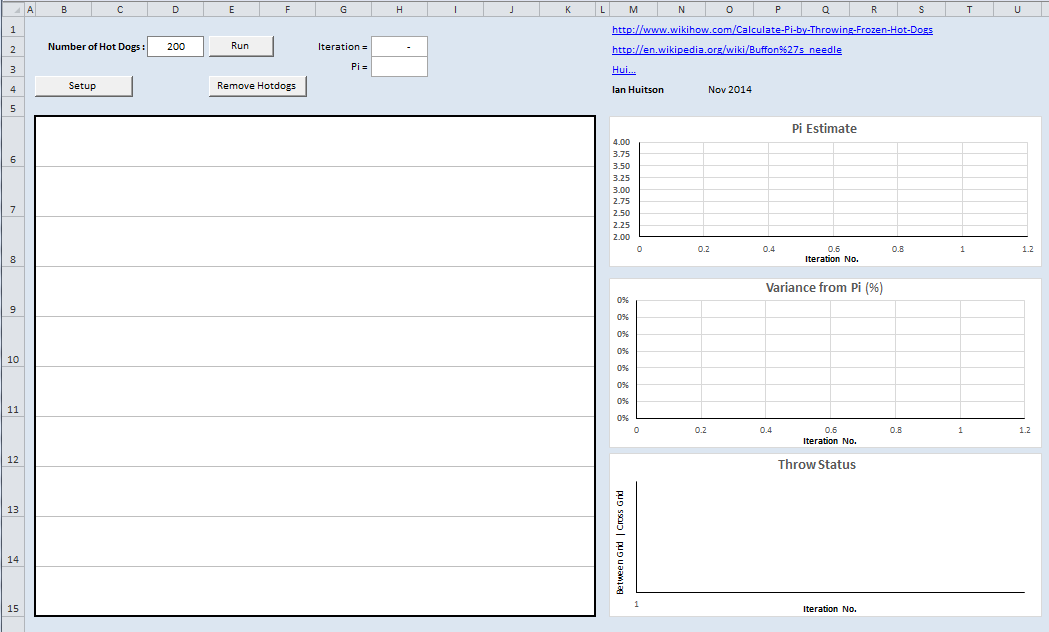Excel doesn't have a Hotdog Drawing tool, but it does have a basic Drawing facility and so a straight line will suffice as a hotdog.
By using a Line I can use VBA to calculate its starting position and rotation as well as the color and line thickness, and then draw the hotdog.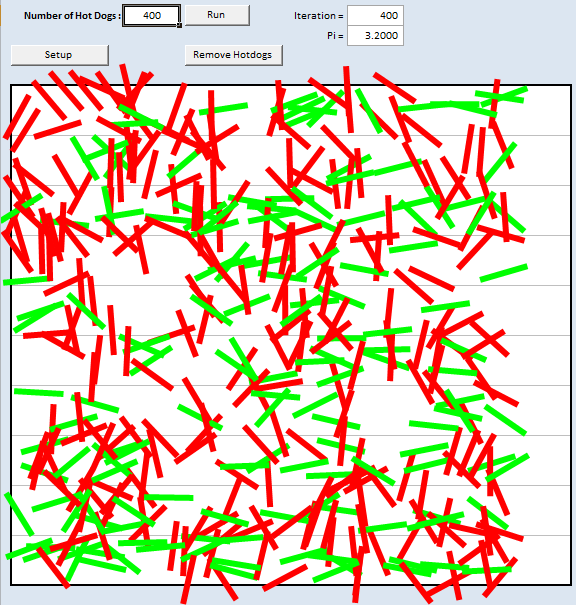It is a shame that although I can manually select a HotDog and change the Line Ends to be round, this functionality hasn't been included into the VBA Object Model and so we are left with straight lines with flat ends as hotdogs.
Maybe they have just been trimmed by the VBA Hotdog vendor?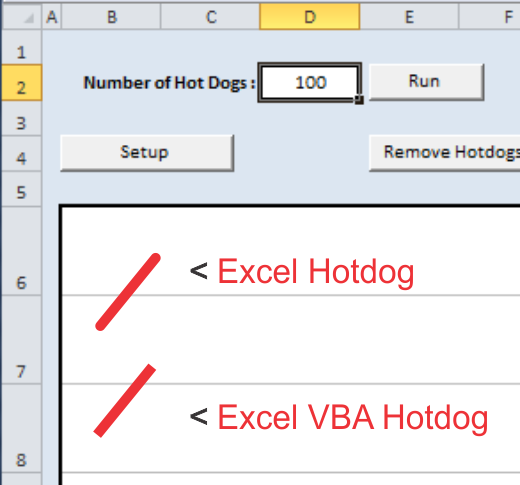Once a hotdog is thrown we need to accumulate some statistics.

Simplistically I only need to know the number of hotdogs that cross/touch a grid line and that could be simply accumulated as each throw occurred.

But once I started I couldn't help myself and added the history and cumulative Pi value and variance from Pi as charts. I also colored the hotdogs according to there location Red for Hotdogs that Crossed a grid line and Green Hotdogs for those that missed a gridline.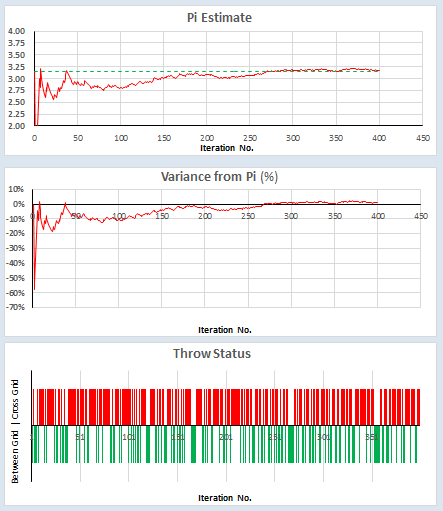The remainder of this post will examine the techniques used for each section.

Simulation
The basic of the simulation are as follows
1. Setup Grid
2. Throw a random Hotdog
a. Choose a random centroid X, Y and Rotation for a Hotdog
b. Calculate X & Y position of each end
c. Check if the Hotdog intercepts a line
d. Draw the HotDog
e. Record the throws status
f. Tally results
3. Repeat step 2
4. Calculate Pi

Of course this could all be done in memory. There is no need to display the hotdogs. Displaying them will definitely slow down the process. But wouldn't that be boring !

Setup a Grid

Excel uses an orthogonal grid starting at 0,0 at the Top Left corner of the A1 Cell. X values increase to the right and Y values increase down the worksheet.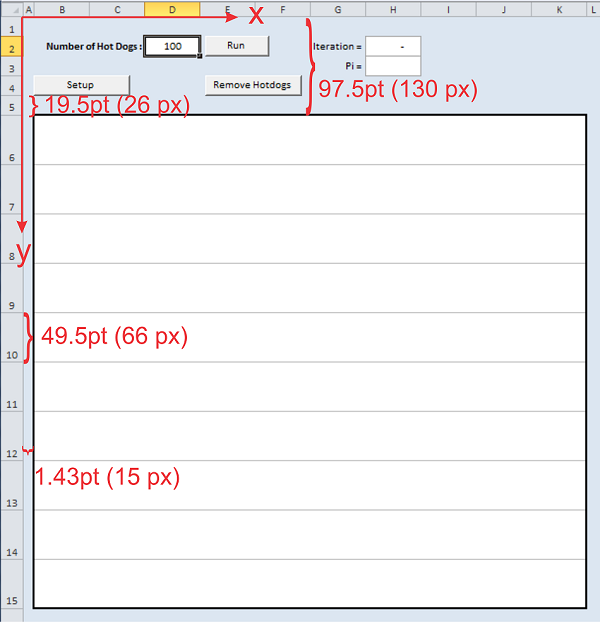I originally decided to have grid of 10 rows of size 50pts, which is 500pts. This allows for space above/below for inputs, controls and stats. I was going to use a Hotdog length of 50.

But in Excel 2010 using a standard Calibri Font, I could only get an odd 49.5 pts Row Spacing (66 pixels). Close enough and I can use that as a variable in VBA when I check the hotdogs location, more on this later.

Setup Code
To ensure the grid is correctly calibrated a small piece of VBA is used to setup the Row sizes

It only need to be used once on a PC or after people change the view zoom or row /column sizes etc, just to make sure everything is setup correctly.

Sub Setup()
Const HotDogLength As Double = 49.5

Worksheets("Throw").Select
Range("A1").Select
Application.ScreenUpdating = False

'Cleanup old hotdogs
CleanUp

'Setup the Throw Sheet
Columns("A:A").ColumnWidth = 1.43
Columns("B:K").ColumnWidth = 10
Rows("1:5").RowHeight = 19.5
Rows("6:17").RowHeight = HotDogLength
Rows("18:1048576").EntireRow.Hidden = True

With Range("B6:K15")
.Borders(xlDiagonalDown).LineStyle = xlNone
.Borders(xlDiagonalUp).LineStyle = xlNone
With .Borders(xlEdgeLeft)
.LineStyle = xlContinuous
.ThemeColor = 2
.TintAndShade = 0
.Weight = xlMedium
End With
With .Borders(xlEdgeTop)
.LineStyle = xlContinuous
.ThemeColor = 2
.TintAndShade = 0
.Weight = xlMedium
End With
With .Borders(xlEdgeBottom)
.LineStyle = xlContinuous
.ThemeColor = 2
.TintAndShade = 0
.Weight = xlMedium
End With
With .Borders(xlEdgeRight)
.LineStyle = xlContinuous
.ThemeColor = 2
.TintAndShade = 0
.Weight = xlMedium
End With

With .Borders(xlInsideVertical)
.LineStyle = xlContinuous
.ThemeColor = 1
.TintAndShade = 0
.Weight = xlMedium
End With
End With

Range("Runs") = 200
Range("Runs").Select
Application.ScreenUpdating = True

End Sub
The Column sizes aren't important but from an aesthetic point of view it looks better if the hotdogs are within a defined area.

Only the Hotdogs Y value is required or used for checking the hotdogs location, This will be discussed later.

The Main Simulation

The Main VBA code runs through the steps of the simulation as described above

2. Throw a random Hotdog
a. Choose a random centroid X, Y and Rotation for a Hotdog
b. Calculate X & Y position of each end
c. Check if the Hotdog intercepts a line
d. Draw the HotDog
e. Record the throws status
f. Tally results

a. Choose a random centroid X & Y position and Rotation for a Hotdog

For each throw of a hotdog we need to calculate a random X, Y coordinate of the centroid of the hotdog and a random rotation of the hotdog between 0 and 2 x Pi Radians

'Calc start pos

Randomize
xc = XOffset + Rnd() * 10 * HotDogLength
yc = YOffset + 10 * HotDogLength * Rnd()
Rot = Rnd() * 2 * Pi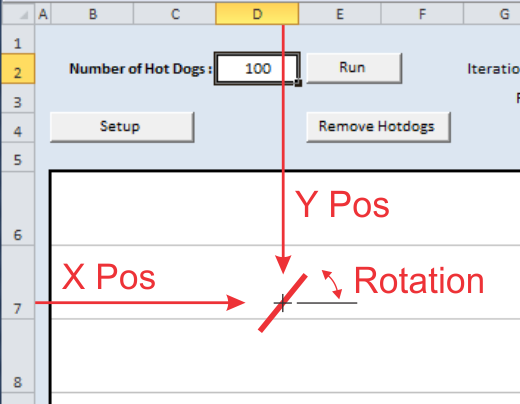b. Calculate X & Y position of each end

From the Centroid, Rotation and Hotdog length we can calculate the end position of each hotdog using simple trigonometry.

'Calc end positions
x1 = xc + Cos(Rot + Pi) * HotDogLength / 2
y1 = yc + Sin(Rot + Pi) * HotDogLength / 2
x2 = xc + Cos(Rot) * HotDogLength / 2
y2 = yc + Sin(Rot) * HotDogLength / 2

c. Check if the Hotdog crosses a gridline

To check if a Hotdog crosses a grid line it is simply a matter of determining which section of the grid each end is in. If they are in different sections the hotdog crosses a line.
This is easily achieved by calculating the Integer value of the hotdogs two end Y values divided by the Grid Spacing. The YOffset is first subtracted to normalise the data .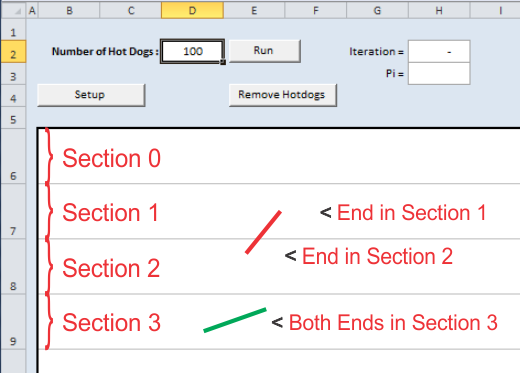If Int((y1 - YOffset) / HotDogLength) = Int((y2 - YOffset) / HotDogLength) Then
Worksheets("Results").Cells(same, 1) = i
HotdogColor = RGB(0, 255, 0) 'Green
Worksheets("Results").Cells(i + 1, 6) = -10
same = same + 1
Else
Worksheets("Results").Cells(cross, 2) = i
HotdogColor = RGB(255, 0, 0) 'Red
Worksheets("Results").Cells(i + 1, 6) = 10
cross = cross + 1
End If

d. Draw the Hotdog

Once the code has determined if the Hotdog is a Red(Crosses a gridline) or Green (Is between the gridlines) we can draw the hotdog. The drawing is outsourced to a small subroutine to simplify the code flow.

Sub DrawDogs(x1, y1, x2, y2, HotdogColor)
'Draw Hotdog
ActiveSheet.Shapes.AddConnector(msoConnectorStraight, x1, y1, x2, y2).Select

With Selection.ShapeRange.Line
.Visible = msoTrue
.Weight = 6
'.capType = msoRound ??? come on MS Guys complete the Object Model
.ForeColor.RGB = HotdogColor
End With
Application.ScreenUpdating = True
End Sub

The function is called by simply calling the DrawDogs subroutine which requires the X & Y values for each end of the dog as well as the Hotdogs color

Call DrawDogs(x1, y1, x2, y2, HotdogColor)

e. Tally results

To calculate Pi using this technique we simply need the tally of the Number of Hotdogs that cross a Gridline and the Total thrown

I decided early on in the design of the workbook that keeping records of which throw was a Cross or Miss may be useful and so 2 columns are setup to store these.

All results are stored on the Results sheet

Columns A & B keep a record of which throw is which type of throw

Column E stores the running Pi and Column F is used for the display of the individual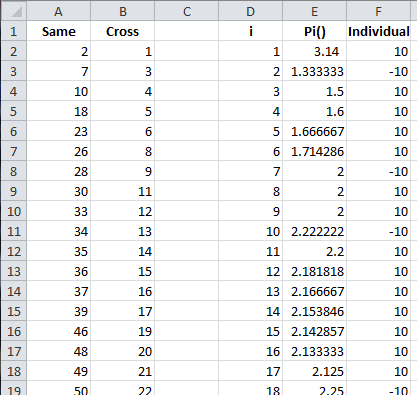Display Results

6 measures are monitored on a throw by throw basis

1. The individual hotdog status as Red (touches a gridline) or Green (sits between gridlines)
2. The Throw No.
3. The Current estimate of Pi
4. The Historical Estimate of Pi vs Pi
5. The Variance from Pi as a percentage of Pi
6. The historical throw Status of each throw as either Cross or Not-Crossed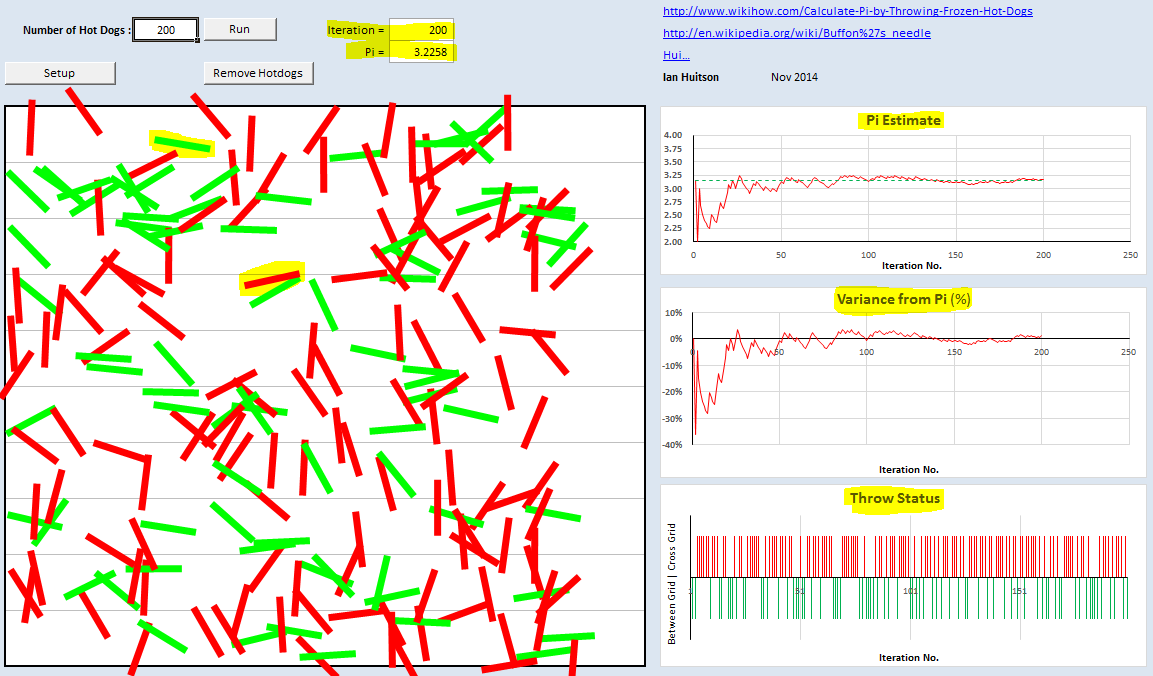A number of Named Formula are used to convert the data to a suitable format for charting.
The Named Formula are then used directly in the various charts.

These include:

 Worksheet Storage Names Runs =Throw!\$D\$2 Iteration =Throw!\$H\$2 Summary Names Cross =COUNT(OFFSET(Results!\$B\$2,,,COUNTA(Results!\$B:\$B)-1,1)) Same =COUNT(OFFSET(Results!\$A\$2,,,COUNTA(Results!\$A:\$A)-1,1)) Calculated Names i =OFFSET(Results!\$D\$1,1,,COUNTA(Results!\$D:\$D)-1,1) PiEst =OFFSET(i,,1) Ind =OFFSET(i,,2) PiAct =PiEst*0+PI() PiVar =(PiEst-PI())/PI()

The above Named Formula are used to transfer the results into the charts on the Throw Worksheet.

Remove Hotdogs

Prior to a simulation run it is good practice to remove the existing hotdogs from the floor and so it is in the Excel implementation.

This is achieved by using a small piece of VBA code.

Sub CleanUp()
Dim Shp As Shape

Worksheets("Throw").Select
For Each Shp In ActiveSheet.Shapes
If Left(Shp.Name, 6) <> "Button" And Left(Shp.Name, 6) <> "Chart " Then
Shp.Delete
End If
Next Shp

Worksheets("Results").Range("A2:B10000").ClearContents
Worksheets("Results").Range("D3:F10000").ClearContents
Worksheets("Results").Range("F2").ClearContents

[Iteration] = 0
[Iteration].Select

End Sub
The code loops through the shapes in the Shapes collection and if not named "Chart" or "Button" deletes it
It also resets the Results sheet.

Download

You can download a copy of
this file which has only been tested in excel 2010 and 2013 from Download File

Closing

I hope you enjoy this implementation in Excel of the Buffon's Needle provided by Hui, proving that indeed Excel can throw Frozen Hotdogs with the best of them.

I have ran this many times with up to 10,000 hotdogs without issue, however from time to time I have experienced an  issue where Chart 3 becomes invisible. If this happens simply close the file without saving and re-open it.
If you work out why please let me know.

About Hui

Hui is a Consulting Mining Engineer by profession and guest author at ExcelHero Blog and Chandoo.org.
Hui received a Microsoft MVP award (Excel) in 2013 and 2014.

Hui has published over 100 posts about all things Excel at:

@ ExcelHero Blog

Motion Induced Blindness

Lilac Chaser

Counter-Intuitive Illusory Contours

M. C. Escher

Two Sinusoids

Cafe Wall

Curry's Paradox

@ Chandoo.org

About Hui
If you liked this article, please share it!## 1 Comment

Woow! Calculating pi is fun with hotdogs haha.

We offer a free dutch excel 2013 course at http://www.learnit.nl/gratiscursus/excel2013/

## Leave a comment

OpenID accepted here Learn more about OpenID### About this Entry

This page contains a single entry by Daniel Ferry published on November 24, 2014 10:31 PM.

Master Excel PowerPivot Now was the previous entry in this blog.

Find recent content on the main index or look in the archives to find all content.